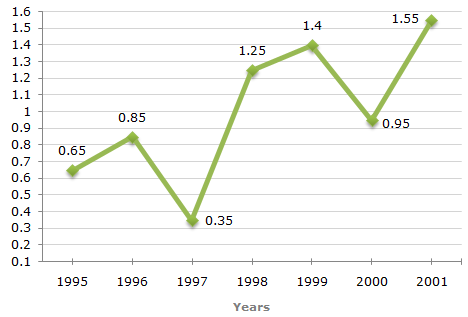# Data Interpretation - Line Charts - Discussion

### Discussion :: Line Charts - Line Chart 2 (Q.No.4)

The following line graph gives the ratio of the amounts of imports by a company to the amount of exports from that company over the period from 1995 to 2001.

Ratio of Value of Imports to Exports by a Company Over the Years.4.

If the imports of the company in 1996 was Rs. 272 crores, the exports from the company in 1996 was ?

 [A]. Rs. 370 crores [B]. Rs. 320 crores [C]. Rs. 280 crores [D]. Rs. 275 crores

Explanation:

Ratio of imports to exports in the year 1996 = 0.85.

Let the exports in 1996 = Rs. x crores.

 Then, 272 = 0.85x = 272 = 320. x 0.85Exports in 1996 = Rs. 320 crores.

 Subha said: (Aug 24, 2016) How did 272 came?

 Ashwini said: (Nov 26, 2017) In question only they have given import of the company in 1996 was 272. And also there is a formula import/export.